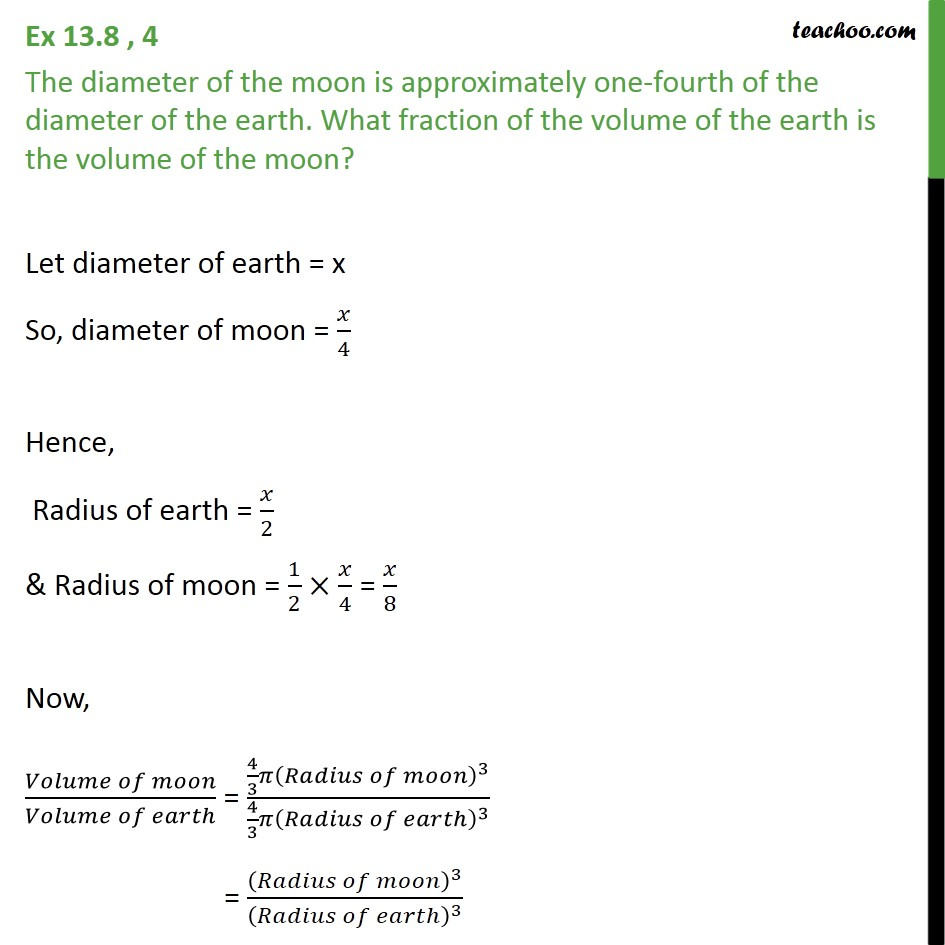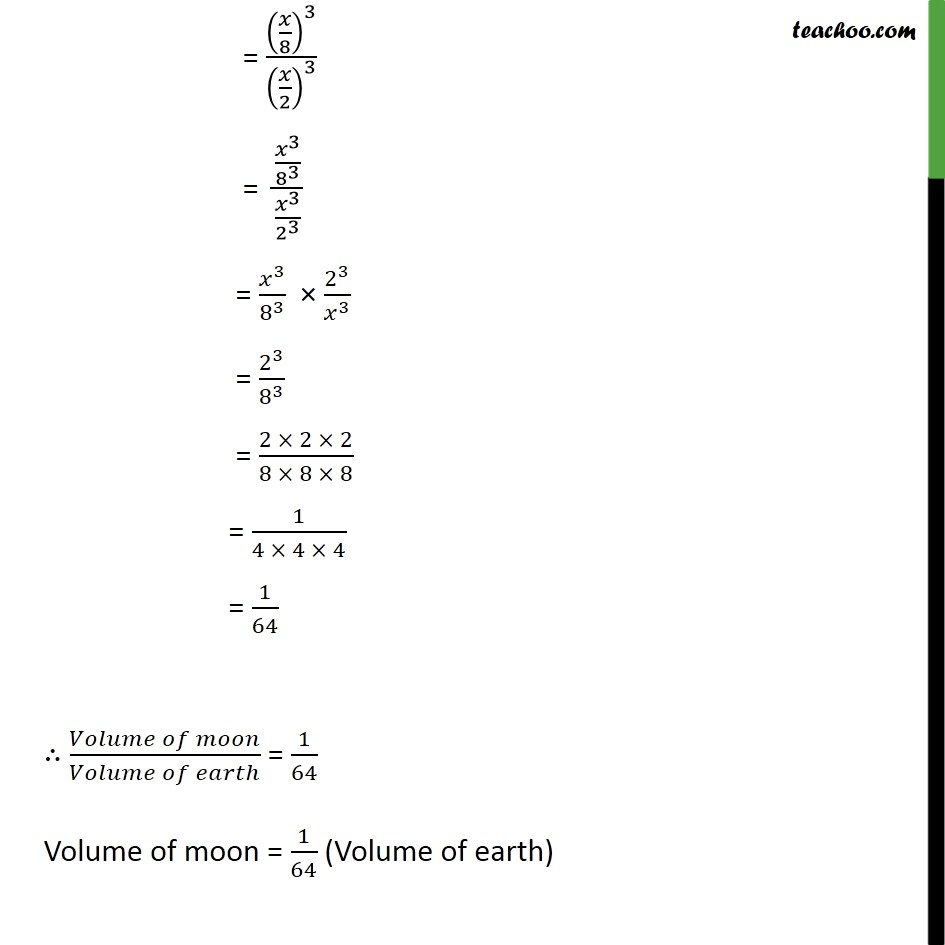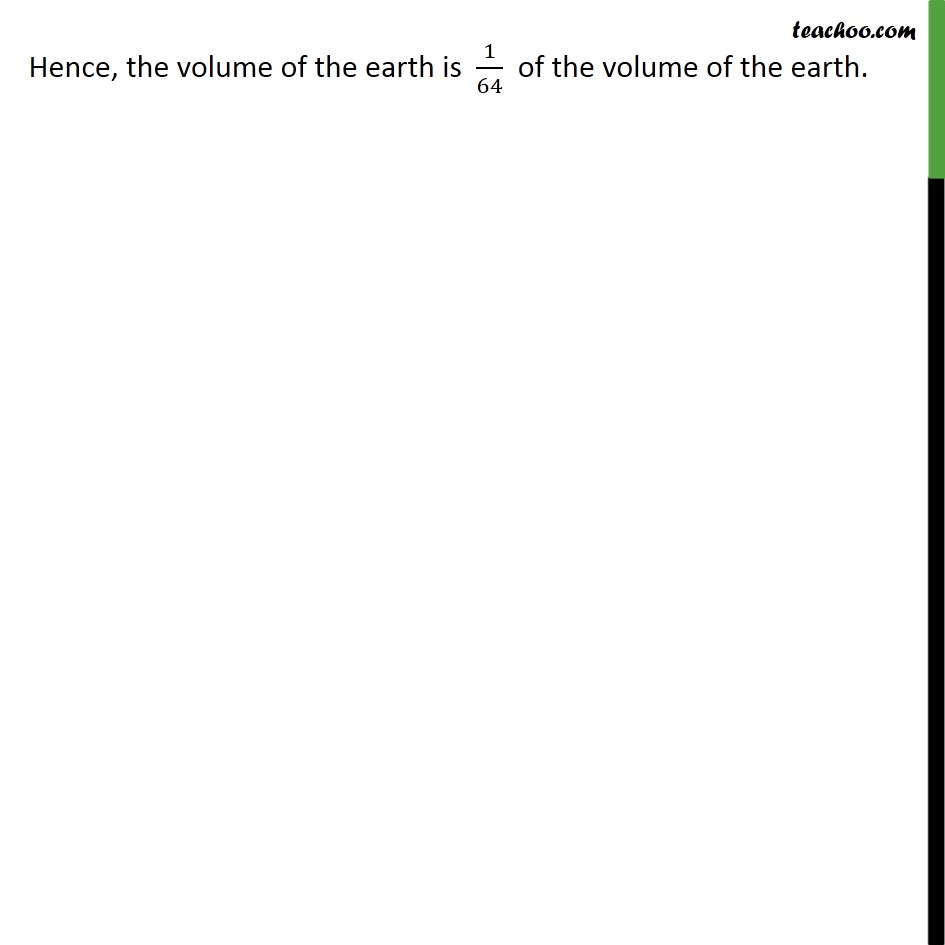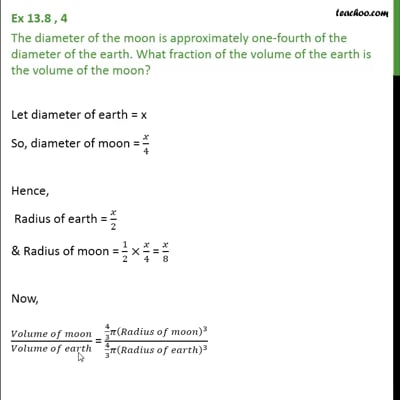Ex 13.8

Chapter 13 Class 9 Surface Areas and Volumes (Term 2)
Serial order wiseThis video is only available for Teachoo black users

### Transcript

Ex 13.8 , 4 The diameter of the moon is approximately one-fourth of the diameter of the earth. What fraction of the volume of the earth is the volume of the moon? Let diameter of earth = x So, diameter of moon = 𝑥/4 Hence, Radius of earth = 𝑥/2 & Radius of moon = 1/2×𝑥/4 = 𝑥/8 Now, (𝑉𝑜𝑙𝑢𝑚𝑒 𝑜𝑓 𝑚𝑜𝑜𝑛)/(𝑉𝑜𝑙𝑢𝑚𝑒 𝑜𝑓 𝑒𝑎𝑟𝑡ℎ) = (4/3 𝜋(𝑅𝑎𝑑𝑖𝑢𝑠 𝑜𝑓 𝑚𝑜𝑜𝑛)^3)/(4/3 𝜋(𝑅𝑎𝑑𝑖𝑢𝑠 𝑜𝑓 𝑒𝑎𝑟𝑡ℎ)^3 ) = (𝑅𝑎𝑑𝑖𝑢𝑠 𝑜𝑓 𝑚𝑜𝑜𝑛)^3/(𝑅𝑎𝑑𝑖𝑢𝑠 𝑜𝑓 𝑒𝑎𝑟𝑡ℎ)^3 = (𝑥/8)^3/(𝑥/2)^3 = (𝑥^3/8^3 )/(𝑥^3/2^3 ) = 𝑥^3/8^3 × 2^3/𝑥^3 = 2^3/8^3 = (2 × 2 × 2)/(8 × 8 × 8) = 1/(4 × 4 × 4) = 1/64 ∴ (𝑉𝑜𝑙𝑢𝑚𝑒 𝑜𝑓 𝑚𝑜𝑜𝑛)/(𝑉𝑜𝑙𝑢𝑚𝑒 𝑜𝑓 𝑒𝑎𝑟𝑡ℎ) = 1/64 Volume of moon = 1/64 (Volume of earth) Hence, the volume of the earth is 1/64 of the volume of the earth.